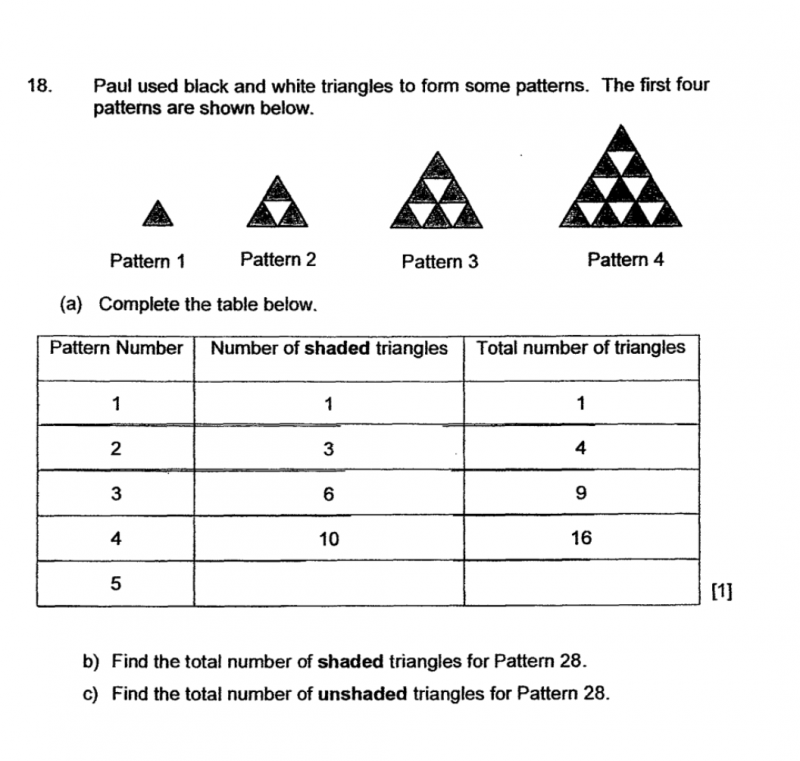# QuestionPoints to note of the pattern 1 to 4 in the table and diagram.
1. Total number of triangle is always squared of Pattern Number
2. Shaded triangles is always ‘plus Pattern number’ more than unshaded. (Add another column for unshaded triangle in the table, you’ll see this clearer).

For pattern 28
Total triangles = 28 X 28 = 784

using Point 2, where shaded is always “+ pattern number” more than unshaded.

Unshaded + (Unshaded +28 ) = 784
2(Unshaded) = 784 – 28 = 756
Unshaded = 756 / 2 = 378 # for answer (c)

Shaded = 378 +28 = 406 # for answer (b)

0 Replies 0 Likes

Haiz … sorry … how u get

28×29/2? Why 29/2?

Math not v good that’s why need step by step guide …

your steps are similar to what is given in the answer key which blurs me.

1 Reply 0 Likes